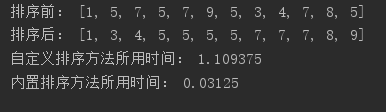# Python实现从键盘接收若干个整数，存放在列表中。自己实现某种排序算法，将输入数据进行升序排列，并将排序结果在屏幕上输出，并对比该算法与列表自带sort方法的性能。

2019-01-03 13:32 344 查看

```#-*-coding:utf_8-*-
import time

#从键盘输入
s=input()
list1=s.split(' ')
list2=[]
for a in list1:
list2.append(int(a))
list3=list2
print("排序前：",list2)

#自定义排序方法
def use_my():
k = len(list3)
for i in range(k - 1):
for j in range(k - i - 1):
if list3[j] > list3[j + 1]:
list3[j], list3[j + 1] = list3[j + 1], list3[j]
use_my()
print("排序后：",list3)
#内置sort方法
def use_sort():
list2.sort()

if __name__ == '__main__':
# 每种方法执行10000次
start=time.process_time()
for i in range(100000):
use_my()
end=time.process_time()
mytime=end-start

start =time.process_time()
for i in range(100000):
use_sort()
end =time.process_time()
sorttime = end - start

print("自定义排序方法所用时间：",mytime)
print("内置排序方法所用时间：",sorttime)```